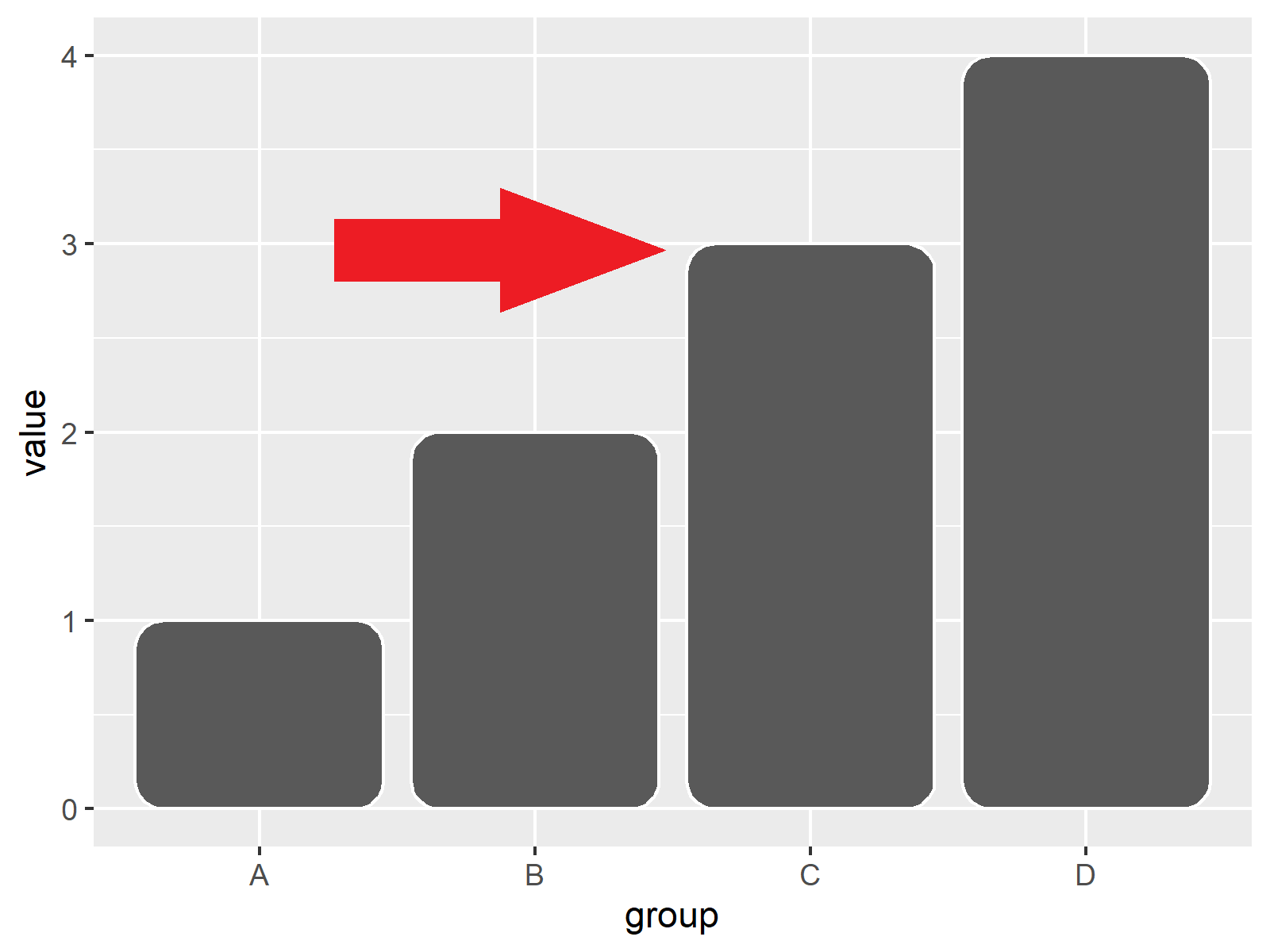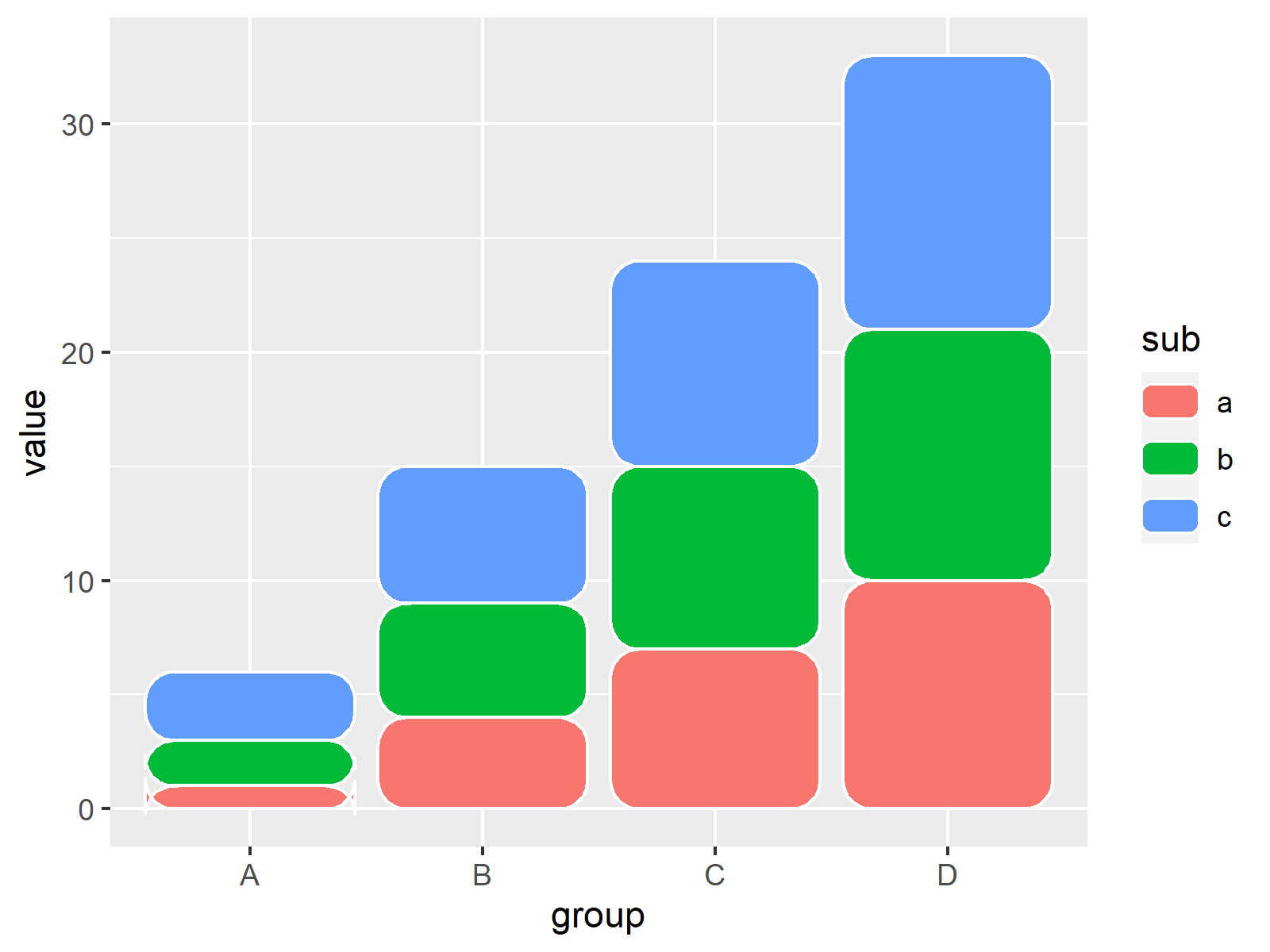# Draw ggplot2 Barplot with Round Corners in R (2 Examples) | ggchicklet Package

In this tutorial, I’ll demonstrate how to create a ggplot2 barchart with rounded bars using the ggchicklet package in the R programming language.

The content of the page is structured as follows:

Let’s do this…

## Introduction to the ggchicklet Package

The ggchicklet package, created and maintained by Bob Rudis, provides functions to create rounded rectangle segmented column charts (i.e. “chicklets”).

## Example 1: Draw ggplot2 Barplot with Round Corners Using ggchicklet Package

In Example 1, I’ll show how to draw barplots with rounded corners using the ggplot2 and ggchicklet packages in R.

For this example, we first have to create some data:

```data1 <- data.frame(value = 1:4, # Create example data group = LETTERS[1:4]) data1 # Print example data```By running the previous code, we have created Table 1, i.e. a data frame containing a numeric and a character column.

Next, we have to install and load the ggplot2 package:

```install.packages("ggplot2") # Install ggplot2 package library("ggplot2") # Load ggplot2 package```

We can now draw a regular ggplot2 barplot using the geom_col function as shown below:

```ggplot(data1, aes(group, value)) + # ggplot2 barplot with regular corners geom_col()```After executing the previous R programming code the barchart visualized in Figure 1 has been created. As you can see, the corners of the bars are not rounded yet.

To create a bargraph with round corners, we have to install and load the ggchicklet software package as well:

```install.packages("ggchicklet", # Install & load ggchicklet package repos = "https://cinc.rud.is") library("ggchicklet")```

Now, we can use the geom_chicklet function of the ggchicklet package to draw a barplot with round corners. Note that we can use the radius argument within the geom_chicklet function to specify how round the corners should be:

```ggplot(data1, aes(group, value)) + # ggplot2 barplot with round corners geom_chicklet(radius = grid::unit(3, "mm"))```As you can see, we have created another ggplot2 barplot, but this time the corners of the bars have been rounded.

## Example 2: Draw Stacked ggplot2 Barplot with Round Corners Using ggchicklet Package

In my opinion, rounded corners look especially petty when drawing stacked barplots.

To illustrate that, we first have to create another data set:

```data2 <- data.frame(value = 1:12, # Create example data group = rep(LETTERS[1:4], each = 3), sub = letters[1:3]) data2 # Print example data```Table 2 shows the output of the previous code: A data frame with a values column as well as group and subgroup columns.

Next, we can use the geom_chicklet function to draw a stacked barplot of these data. Note that we are also specifying the fill argument within the aesthetics of our plot:

```ggplot(data2, aes(group, value, fill = sub)) + # Stacked barplot with round corners geom_chicklet(radius = grid::unit(3, "mm"))```The previous R syntax has produced Figure 3, i.e. a stacked barchart with round corners.

## Video, Further Resources & Summary

Do you want to learn more about the creation of a ggplot2 barchart with rounded bars using the ggchicklet package? Then I can recommend watching the following video on my YouTube channel. I show the content of this article in the video.

Furthermore, you might want to read the other tutorials on statisticsglobe.com.